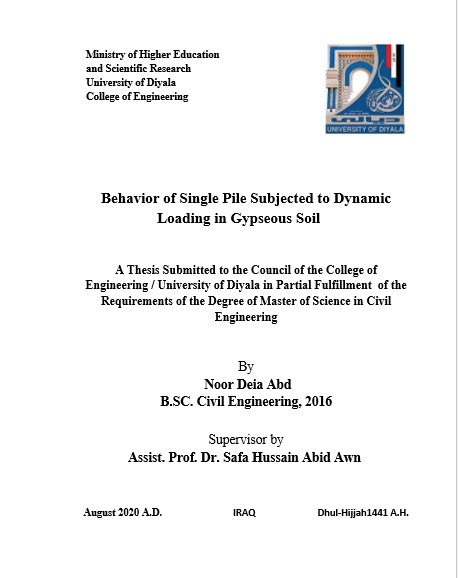## رسالة ماجستير / نور ضياء

Abstract

In this thesis, the dynamic response of single pile with a static load is studied under the dynamic load that were generated by use of an electric rotary motor with eccentric loading installed above cap pile, the tests are performed under dry and soaked states.                                          .
Many parameters are taken into consideration, including slenderness ratio (L/D) 12, 17, 22, 27 for solid steel pile with frequency 10 Hz and gypsum content 30%, different frequencies 10, 15, 20, 25 for solid steel pile with (L/D) 27, gypsum content 65% for solid steel pile, also (L/D) 27 and frequency 10 Hz, types of pile (concrete pile, hollow steel pile, rough steel pile, timber pile) with (L/D) 27  and frequency 10 Hz in all tests.                   .                                                                                                                         .      The results of the tests showed that the velocity of vibration, acceleration of pile, displacement amplitude and settlement decrease with an increase in slenderness ratio and decrease in soaked soil compared to the corresponding values in dry soil where velocity decreased when increasing the slenderness ratio from 12 to 27 in the dry and soaked states by 78.2% and 77.2% respectively. Acceleration decreased by 84% and 80%, respectively, while the displacement amplitude decreased by 66.67% and 61.4%, respectively.  As well, settlement decreased by 31.25%.                               .

The tests also showed that the velocity of vibration, acceleration, displacement amplitude of the solid steel pile with L/D 27 constant in all tests increased with increasing the frequency of the vibration source as the velocity decreased when the frequency is reduced from 25 to 10 Hz in the dry and soaked condition by 98% and 97.8% respectively, acceleration decreased by 98% and 97.8% respectively. While the displacement amplitude decreased by 84.7% and 80% respectively and the settlement is decreased by 31.25%.                                                                               .       .
In addition, the results of tests showed that the displacement amplitude, velocity of vibration and acceleration increased with an increase in the gypsum content in soil, as the velocity decreased when decreasing the gypsum content from 65% to 30%  in the dry and soaked states by 58.85% and 50% respectively. Acceleration decreased by 29.4% and 20%, respectively. While the displacement amplitude decreased by 20% and 30.5%, respectively and settlement decreased by 12%.                                  .
The results also showed that the velocity of vibration, acceleration and displacement amplitude are differed according to the type of pile. The dynamic response showed that an increase in timber pile compared to other type due to low hardness, while the settlement is less than in other piles as a result of friction between pile and soil.